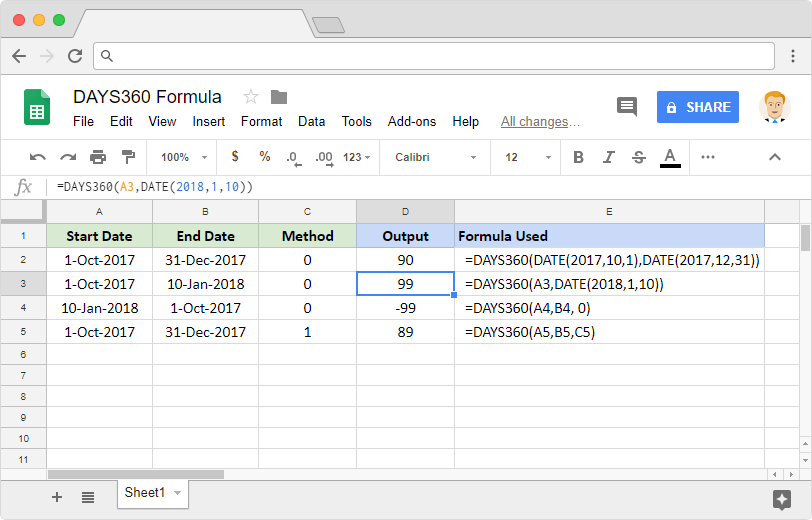# How to use the DAYS360 Google Sheets formulaLet’s say we need to know how far two different dates are separated from each other, based on the 360 day year. Probably for the purposes of some financial interest calculations. We can make use of the DAYS360 formula in Google Sheets for this purpose. Please note that formula is supposedly built for use in a financial setting. Using this in any other context may result in incorrect output values.

### Syntax

DAYS360(start_date, end_date, [method])

• start_date – the date using which the formula calculates the ‘date difference’ from. This input has to be date type data, which we can ensure either by using DATE type returning functions or by using references to the cells that have dates.
• end_date – the date until which the formula calculates the ‘date difference’ to. Just like the start_date, this too has to be date type data.
• method – [ OPTIONAL – 0 by default ] – a 0 indicates that the DAYS360 formula should use US method of day count. Any other value indicates that the formula should use European method.

The US method, there are a couple scenarios to consider. If the start_date is the last day of a month, the formula changes it to 30 for the purposes of the calculation. If the end_date is the last day of a month and start_date is earlier than the 30th of that month, then the formula changes the end_date to the first day of the following month. Otherwise, the formula considers the end_date as 30th of that month.

With the European method, it is relatively simple. If any start_date or end_date falls on the 31st of a month, then the formula changes it to 30th of that month.

### Usage: DAYS360 formula in Google Sheets

We just learned the way the syntax of the DAYS360 formula works. It is time to dive head-first into applying this practically on the Google Sheets application. Please have a look at the snapshot below.In the first example, we notice the output is 90. But if we calculated the actual difference using the DATEDIF formula, it will give us 91. So, this clearly demonstrates that the financial context to calculate how far two dates are from each other is different from the actual context.

While the initial three example formulas used the US method of day counting, the final formula uses the European method.

We encourage you to flex your thinking muscles and analyze how each example formula calculated the difference. You might as well compare each of these with the DATEDIF application to observe the deviations (if any).